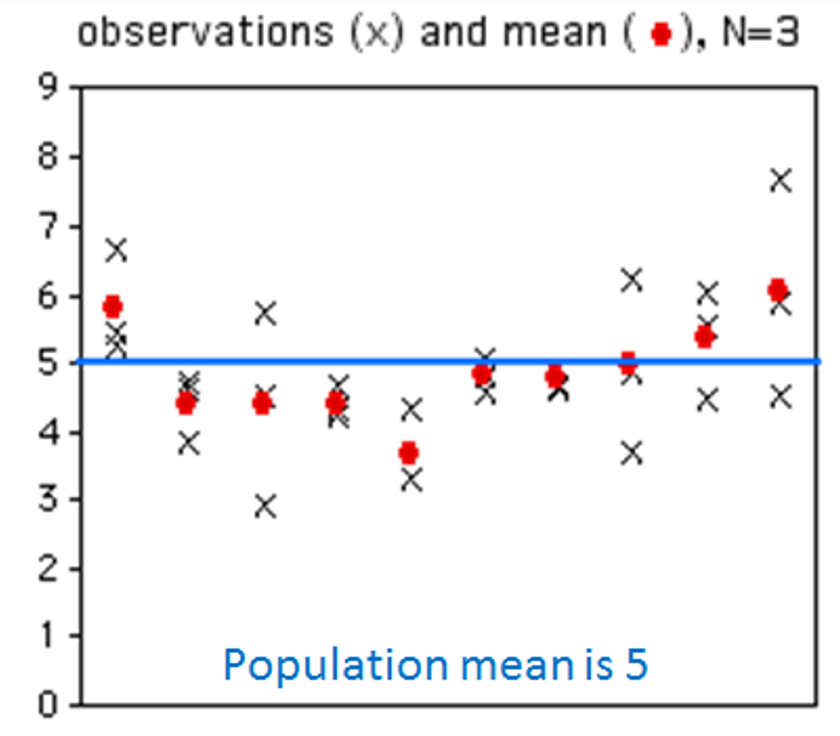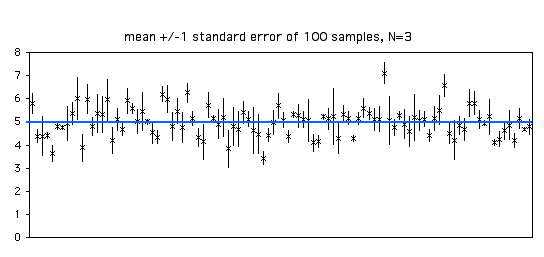# Standard Error

(8 minutes)

## Introductions

• As needed ... Introduce yourselves!

• Icebreaker: What is a good non-computer game to play over Zoom?

## Groupwork

For each question:

### Probability Distributions

Consider the below pictures. On the x-axis is the experiment number and the y-axis is the value. The blue lines depict the population mean. Each x is a sample value.The first shows 10 experiments each `N=3`, with the experimental mean shown with a red dot.

The second shows 100 experiments with the bars depicting a computation of the standard error for that experiment.

1. How many of the standard error bars should intersect the blue line?

2. What would the graphs look like if `N = 100`?

3. If `N = 100`, how many of the standard error bars should intersect the blue line?

## Hand-in

Have ONE person from each group submit the group's answers:

https://wpi.qualtrics.com/jfe/form/SV_b7pJhFXcwPj7U6W

Make sure to include the names of all group members.

Happy standard error-ing!

-- Mark